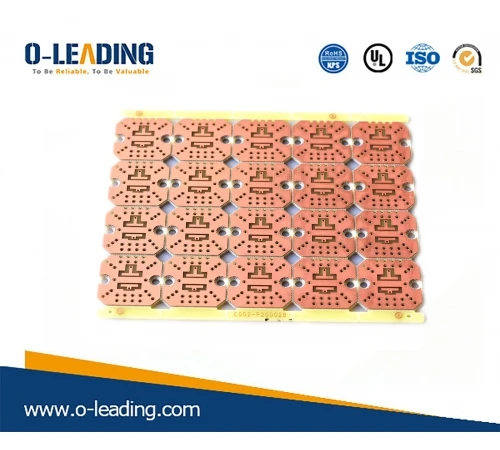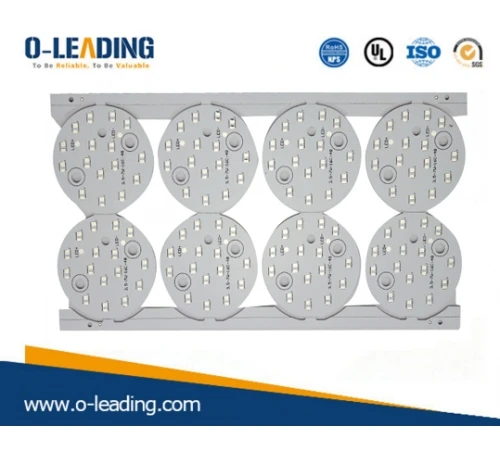Home > News > PCB News > The operating principle and the variable frequency varistor function
TEL: + 86-13428967267

FAX: + 86-4008892163-239121

+ 86-2028819702-239121

Email: sales@o-leading.comCertifications
New Products
Electronic album# The operating principle and the variable frequency varistor function

A variable frequency varistor is a three-phase reactor in which a piece of cast iron or a steel plate is rolled into a core and a winding is wound outside, and is connected to the rotor winding circuit, and the equivalent impedance of the winding reactance and the core loss are determined by the rotor current. The frequency changes.Overview
A variable frequency varistor is a three-phase reactor in which a piece of cast iron or a steel plate is rolled into a core and a winding is wound outside, and is connected to the rotor winding circuit, and the equivalent impedance of the winding reactance and the core loss are determined by the rotor current. The frequency changes.

Since the 1960s, the frequency-sensitive varistor has been widely used to replace the starting resistance to control the start of the asynchronous wound rotor motor. A variable frequency varistor is a static, non-contact electromagnetic component that automatically varies in varying degrees to its frequency sensitivity. The variable frequency varistor is essentially a three-phase reactor with a large loss of iron and its structure is similar to a three-phase transformer without secondary winding.
The variable frequency varistor is a new type of non-contact component with a unique structure. Its external structure is similar to that of a three-phase reactor, ie it has three iron core columns and three windings. The three windings are connected in a star shape and are connected to the wound of the three-stage rotor of the wound motor through a sliding ring and a brush.

When the winding motor starts, the motor speed is very low, so the frequency of the rotor f2 is very large (near f1), and the loss in the core is very large, ie the equivalent resistance Rm is large, thus limiting the current start and increasing initial Pair. As n increases, the frequency of the rotor current decreases (f2 = s f1) and Rm decreases, keeping the starting current and the torque at a certain value. The sensitive frequency varistor effectively uses the uniform variation of the rotor frequency f2 to achieve a uniform reduction in the total resistance of the rotor circuit. After start-up, the rotor windings are short-circuited and the frequency-sensitive varistor is removed from the circuit. Since the equivalent resistance Rm and the Xm reactance of the frequency-sensitive varistor vary with the frequency of the rotor current, the reaction is sensitive, so it is called a frequency-sensitive varistor.principle of operation
The variable frequency varistor is actually a special three-phase iron core reactor, which has a three-column iron core with one winding on each column and the three-phase windings are generally star-shaped. The impedance of the frequency-sensitive varistor changes significantly with the change in the current frequency. When the current frequency is high, the impedance value is also high and when the current frequency is low, the impedance value is also low. This frequency characteristic of the variable frequency varistor is very suitable for controlling the starting process of an asynchronous motor. At start-up, the current frequency of the rotor fz is maximum. Rf and Xd are the largest and the motor can get a high starting torque. After starting, as the rotor speed increases, the frequency of the rotor current gradually decreases and both Rf and Xf decrease automatically. Therefore, the motor can obtain approximately the constant torque characteristic and realize the gradual motor start-up. At the end of the start-up, the frequency varistor must be short-circuited.

effect
A variable frequency varistor is a non-contacting electromagnetic component equivalent to an equivalent impedance. During the motor starting process, since the equivalent impedance decreases automatically with the decrease of the high frequency component in the starting current of the rotor to obtain an automatic varistor, the motor can be started fluidly using only the primary varistor.

The varistor is essentially a three-phase reactor with a very high core loss. It consists of two main parts: an iron core and a coil that are rolled with different steel sheets in the shape of E. A gasket is placed between the steel plates to maintain the distance between the plates for heat dissipation.
When the winding motor starts, the motor speed is very low, so the frequency of the rotor f2 is very large (near f1), and the loss in the core is very large, ie the equivalent resistance Rm is large, thus limiting the current start and increasing initial Pair. As n increases, the frequency of the rotor current decreases (f2 = s f1) and Rm decreases, keeping the starting current and the torque at a certain value. The sensitive frequency varistor effectively uses the uniform variation of the rotor frequency f2 to achieve a uniform reduction in the total resistance of the rotor circuit. After start-up, the rotor windings are short-circuited and the frequency-sensitive varistor is removed from the circuit. Since the equivalent resistance Rm and the Xm reactance of the frequency-sensitive varistor vary with the frequency of the rotor current, the reaction is sensitive, so it is called a frequency-sensitive varistor.Problem
The variable frequency varistor is connected to the tap 90% of the coil when shipped from the factory. If the motor is connected to the varistor, one of the following conditions can be adjusted as follows:

1. The starting current is too big (more than 2.5 times), the start is too fast, you can try to increase the number of turns and connect the tap to 100% of the number of revolutions. The effect is to reduce the starting current and reduce the initial torque.

2. The starting current is too small (less than 2 times). The initial torque is not enough. The boot is too slow. Try to reduce the number of turns and connect the tap to 80% of the turns. The effect is to increase the starting current and increase the initial torque.

3. If the machine is restarted after a period of inactivity, the mechanical load is very heavy and if it is difficult to restart, the motor can be moved several times, so that the machine can be used normally after several rotations.